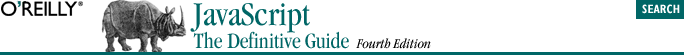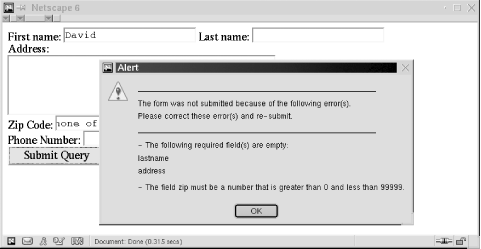home | O'Reilly's CD bookshelfs | FreeBSD | Linux | Cisco | Cisco Exam## 15.4. Form Verification Example

We'll close our discussion of forms with an extended example that demonstrates several of the concepts we've been discussing. Example 15-2 shows how you might use the onsubmit event handler of the Form object to perform input validation so that you can notify the user and prevent the form from being submitted when it contains missing or invalid data. After studying this example, you may want to turn back to Example 1-3, the form-programming example we began this book with. The code of that example probably makes more sense now that you are a JavaScript expert!

Figure 15-2 shows an example form that uses this verification scheme, with the error message that is displayed when the user attempts to submit the form before correctly filling it in.#### Example 15-2. Performing form validation

```<script language="JavaScript1.1">
// A utility function that returns true if a string contains only
// whitespace characters
function isblank(s) {
for(var i = 0; i < s.length; i++) {
var c = s.charAt(i);
if ((c != ' ') && (c != '\n') && (c != '	')) return false;
}
return true;
}

// This is the function that performs form verification. It is invoked
// from the onsubmit event handler. The handler should return whatever
// value this function returns.
function verify(f) {
var msg;
var empty_fields = "";
var errors = "";

// Loop through the elements of the form, looking for all Text and
// Textarea elements that don't have an "optional" property defined.
// Then check for fields that are empty and make a list of them. Also, if
// any of these elements have a "min" or a "max" property defined, verify
// that they are numbers and are in the right range. If the element has a
// "numeric" property defined, verify that it is a number, but don't check
// its range. Put together error messages for fields that are wrong.
for(var i = 0; i < f.length; i++) {
var e = f.elements[i];
if (((e.type == "text") || (e.type == "textarea")) && !e.optional) {
// First check if the field is empty
if ((e.value == null) || (e.value == "") || isblank(e.value)) {
empty_fields += "\n          " + e.name;
continue;
}

// Now check for fields that are supposed to be numeric
if (e.numeric || (e.min != null) || (e.max != null)) {
var v = parseFloat(e.value);
if (isNaN(v) ||
((e.min != null) && (v < e.min)) ||
((e.max != null) && (v > e.max))) {
errors += "- The field " + e.name + " must be a number";
if (e.min != null)
errors += " that is greater than " + e.min;
if (e.max != null && e.min != null)
errors += " and less than " + e.max;
else if (e.max != null)
errors += " that is less than " + e.max;
errors += ".\n";
}
}
}
}

// Now, if there were any errors, display the messages, and
// return false to prevent the form from being submitted.
// Otherwise, return true.
if (!empty_fields && !errors) return true;

msg  = "_____________________________________________________  _\n\n"
msg += "The form was not submitted because of the following error(s).\n";
msg += "Please correct these error(s) and re-submit.\n";
msg += "_____________________________________________________  _\n\n"

if (empty_fields) {
msg += "- The following required field(s) are empty:"
+ empty_fields + "\n";
if (errors) msg += "\n";
}
msg += errors;
return false;
}
</script>

<!------------------------------------------------------------------------
Here's a sample form to test our verification. Note that we
call verify( ) from the onsubmit event handler and return whatever
value it returns. Also note that we use the onsubmit handler as
an opportunity to set properties of the Form objects that verify( )
requires for the verification process.
-------------------------------------------------------------------------->
<form onsubmit="
this.firstname.optional = true;
this.phonenumber.optional = true;
this.zip.min = 0;
this.zip.max = 99999;
return verify(this);
">

First name: <input type="text" name="firstname">
Last name: <input type="text" name="lastname"><br>